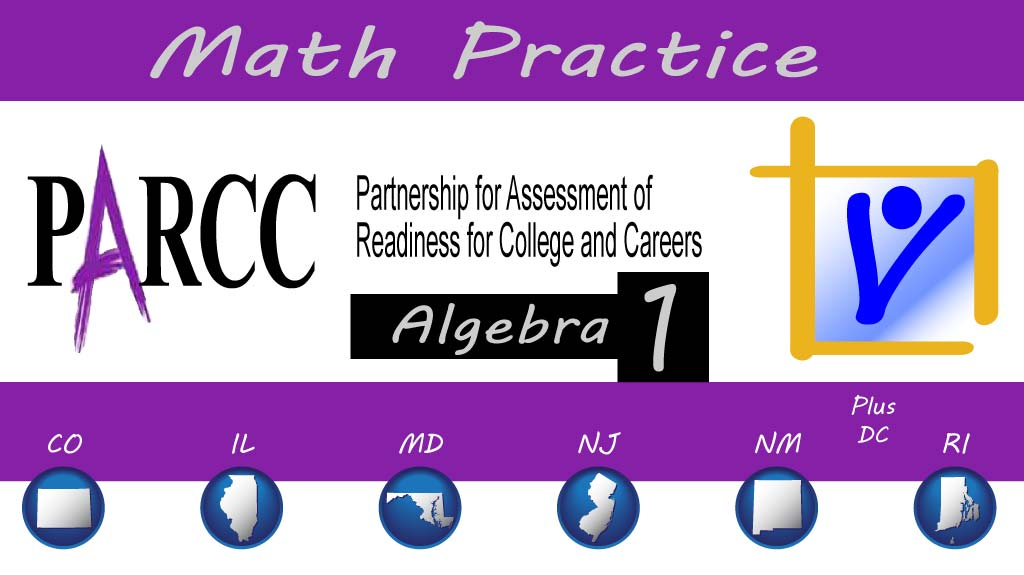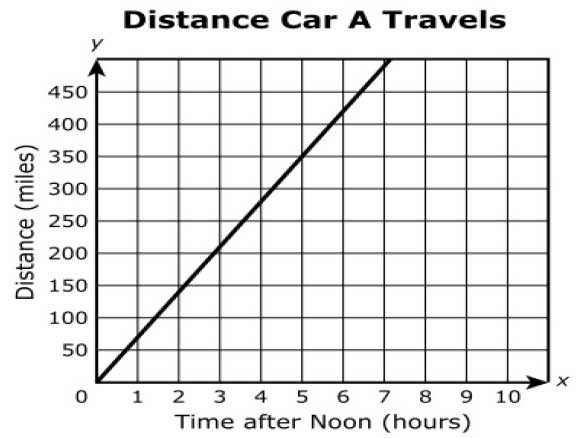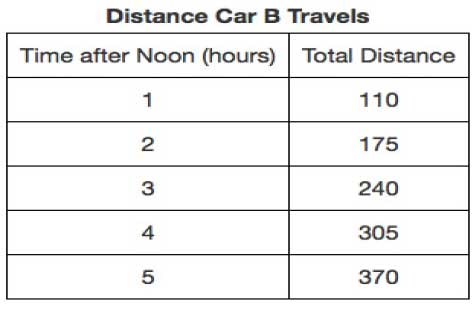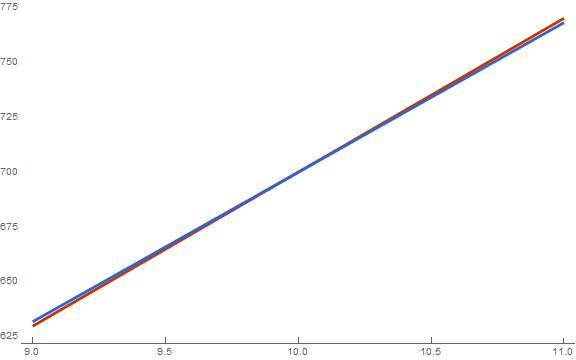Thursday, December 12, 2019# Algebra 1 PARCC: racing cars

#### The following constructed-response question, explained here in hopes of helping algebra 1 students and their parents in Maryland and Illinois prepare for the PARCC test near the end of this school year, appears on the released version of PARCC’s Spring 2016 test for algebra 1 (#30):Three cars are traveling to the same campground along the same route. The cars began at the same place but at different times. Car A began the trip at noon, and cars B and C began the trip at different times before noon. The miles traveled by cars A, B, and C are represented respectively, by the following graph, table, and equation.

### Car A### Car B### Car C$D = 68t + 20$

where D is the total distance, in miles, traveled by car C, and t is the number of hours after noon.

At what time, if ever, will car A pass each of the other cars? Describe any assumptions you made and justify your answer.Correct answer: Car A will overtake car B at 9 PM and car C at 10 PM.

Common Core Math, Reasoning based on securely held knowledge:

The question makes use of eighth-grade math standards, including 8.EE.B: “Understand the connections between proportional relationships, lines, and linear equations.” That standard requires students by the end of eighth grade to “graph proportional relationships, interpreting the unit rate as the slope of the graph. Compare two different proportional relationships represented in different ways. For example, compare a distance-time graph to a distance-time equation to determine which of two moving objects has greater speed.”

However, the question, directed at algebra 1 students, is actually just assuming they already know that and can now use that knowledge to make an argument about mathematics. In other words, the PARCC test sort of requires students to revisit eighth-grade knowledge from a more mature reasoning perspective. They “construct, autonomously, chains of reasoning that will justify or refute propositions or conjectures about linear equations in one or two variables.”

Solution strategy (there are others)

Determine the time (x coordinate on a graph like the one for car A) when car A will meet car B and car C by finding the intersection on the graph.

We are given a graph for car A. The way to find the slope of a graph is by finding a point on the line that exactly crosses at the intersection of two grid lines. Here, the graph for car A seems to go through the point (0, 0) and the point (5, 350).

Since the y axis is in miles and the x axis is in hours, the slope of the line is in “miles per hour” and is determined by finding the rate of change:$\textrm{slope} = \frac{\Delta y}{\Delta x} = \frac{350-0}{5-0} = 70\textrm{mph}$

Since the y intercept is 0, the equation for car A is$\textrm{Car A: } D = 70t + 0$

For car B, we are given a table, but it looks like the mileage traveled between hour 1 and hour 2 is equal to the mileage traveled between hours 3 and hour 4, and all the way down the table. Car B seems to be traveling at a constant speed of 65 mph and started at 1:00 (t = 1), having already traveled 110 miles. Therefore, assuming it was the same speed before that, it started at the 45-mile position. That means the equation for the line for car B would be$\textrm{Car B: } D = 65t + 45$

For car C, we already have an equation:$\textrm{Car C: } D = 68t + 20$

Will car A ever overtake car B? If so, it will occur when the distances are equal and the time is the same. So, just set car A’s distance equal to car B’s distance and solve for the time:$70t + 0 = 65t + 45$$5t = 45$$t = 9$

Since t is the time after noon, car A will meet car B nine hours after noon, or at 9 PM. That makes sense, because car B had a head start (45 miles) and car A was only traveling 5 mph faster than car B. In order to make up that 45 miles, it will take 9 hours.

Will car A ever overtake car C? That would also occur where the distances are equal:$70t + 0 = 68t + 20$$2t = 20$$t = 10$

So since t is the hours after noon, car A will overtake car C at 10 PM the same day. The speeds of these two cars are even closer than car A was to car B, but car C didn’t have quite as much of a head start on car A, being only 20 miles ahead when car A got going. To make up that 20 miles, even at only 2 mph faster, is only going to take 10 hours.

To check, we can plot the different functions and show the different cars meeting at the times found. Because the lines are so close together, we’re going to plot each car individually with car A.Car A is red, and car C, traveling at nearly the same speed, is blue.

We did the same for car B. So, as found in the equation solving and verified in the graphs, assuming the cars have enough gas to maintain a constant velocity, as the problem seems to set up, car A will eventually overtake both car B and car C.

## Analysis of this question and online accessibility

The question is valid in that it tests students’ ability to construct a chain of reasoning about a mathematical scenario.

The question can be delivered online or on paper. However, because students cannot draw using the online tool and will be forced to use words, those who take the test online may be at a disadvantage with this question if they are accustomed to solving or explaining their solution to problems like this using diagrams or graphs. This solution strategy isn’t the only one available, though, but the PARCC test, with the “equation editor” tool, unnaturally forces students’ hand: they must explain their solution in paragraph form, not by laying out a table or drawing a model of the cars, if they are taking the test online.

This means validity and reliability measures for the item may suffer from the different test-taking environment, in terms of responding to the question, experienced by students who take the test on a keyboard and those who take it freehand.

No special accommodation challenges can be identified with this question, so the question is considered fair.

(Minor editorial concerns remain. The question is wordy. The question switches from the present tense to the past tense without any transition: the verb “began” is somewhat jarring, since the question starts out with the present tense verb “are traveling.” The comma before, but not after, the word “respectively” reflects inconsistent style and usage. The use of the word “respectively” is unnecessary since the representations are labeled and adds to the number of words students have to read. The passive voice in the final sentence before the graph is clumsy.)

## Resources for further study

Ron Larson, Laurie Boswell, Timothy D Kanold, Lee Stiff. Algebra 2, Illinois edition. Evanston, Ill.: McDougal Littell, a division of Houghton Mifflin Company, 2008. The book is used in several algebra classes taught in Illinois high schools.

Foerster, Paul A. Algebra and Trigonometry: Functions and Applications, revised edition. Addison-Wesley, 1980, 1984. The book is used in several algebra classes taught in middle and high schools in both Illinois and Maryland.

## Purpose of this series

To help algebra 1 students and their parents prepare for the PARCC test in math, as administered in at least six states, or to just master content on that test, we provide an analysis of every algebra 1 math problem PARCC released in 2016. The series can be found here.Paul Katula is the executive editor of the Voxitatis Research Foundation, which publishes this blog. For more information, see the About page.

### Girls’ volleyball champs in Illinois

We congratulate the Illinois state champions in girls' volleyball: Newark, St Teresa, Sterling, & Benet Academy.

### A weekend of ‘band geeks’ across America

The musical Band Geeks was in performance at a MD high school, just as marching bands from across America named a national champion.

### 2 dead, 3 wounded in Calif. school shooting

Another school shooting has resulted in the death of 2 California high school students. The suspect shot himself and is in custody.

### Mercury makes a transit; next in 2032

A transit of Mercury occurred today and was visible from the US, provided you had sunny skies. It was one of longest possible transits.

### On the Naperville BWW racist incident

A racist incident at a Naperville, IL, sports bar indicates that the threads of racism are strong, perhaps as strong as ever.

### IL bill could excuse absences to vote

A proposed law in IL could give students up to two hours during the school day so they could vote in the upcoming election.

### Loan forgiveness gains some bipartisan support

One Republican from GA, who used to work under Betsy DeVos at the US Education Dept, offers a plan to forgive some student loan debt.

### A band teacher is IL Teacher of the Year

IL named a band teacher the 2020 Teacher of the Year on Oct. 19. He individualizes music instruction and shares his work with 1000s.

### ‘Little Shop of Horrors’ bookends Halloween

Several high schools have decided to add a little spook to their musical stages in this season of Halloween. Music makes it happen.

### New IL law ensures inclusion of LGBTQ+

A law will take effect next school year in IL that will require students to study LGBTQ history as part of the social studies curriculum.

### MoCo doubles down on summer learning loss

Research is at least equivocal about summer learning loss, but maybe there's something to a new plan in Montgomery County, Md.